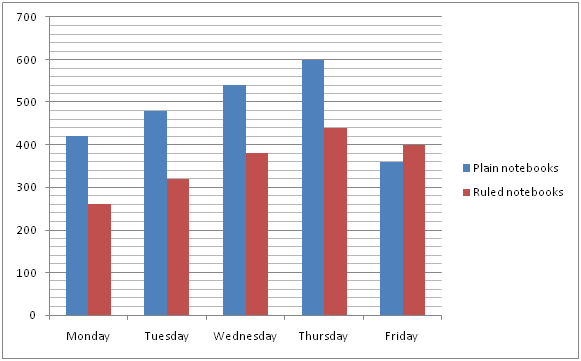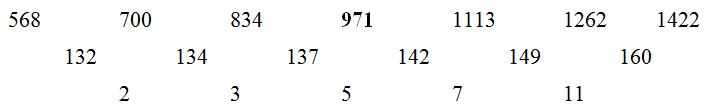# SBI PO Prelims Quantitative Aptitude Questions 2019 (Day-47)

Dear Aspirants, Our IBPS Guide team is providing new series of Quantitative Aptitude Questions for SBI PO 2019 so the aspirants can practice it on a daily basis. These questions are framed by our skilled experts after understanding your needs thoroughly. Aspirants can practice these new series questions daily to familiarize with the exact exam pattern and make your preparation effective.

[WpProQuiz 6364]

### Click Here for SBI PO Pre 2019 High-Quality Mocks Exactly on SBI Standard

Directions (Q. 1 – 2) In the following questions, two equations I and II are given. You have to solve both the equations and give answer as,

a) If x > y

b) If x ≥ y

c) If x < y

d) If x ≤ y

e) If x = y or the relation cannot be established

1) I) 2x2 – 17x + 33 = 0

II) 3y2+y – 52 = 0

2) I) 5x – 7y + 3 = 2x – 3y

II) 5x – 3y + 16 = 0

Directions (Q. 3 – 5): Find the wrong term in the following number series?

3) 13, 17, 43, 147, 605, 3061

a) 147

b) 43

c) 3061

d) 605

e) 13

4) 41, 20, 21, 30, 62, 152.5, 460.75

a) 30

b) 62

c) 41

d) 460.75

e) 20

5) 568, 700, 834, 972, 1113, 1262, 1422

a) 1422

b) 972

c) 700

d) 1262

e) 568

Directions (Q. 6 – 10): Study the following information carefully and answer the given questions.

The following bar graph shows the total number of notebooks (Plain + Ruled) sold by a shopkeeper in 5 different days.6) Find the difference between the total number of notebooks sold on Monday to that of Friday?

a) 100

b) 80

c) 120

d) 60

e) None of these

7) Total number of notebooks sold on Tuesday is approximately what percentage of total number of notebooks sold on Saturday, if the total number of notebooks sold on Saturday is 40 % more than the total number of notebooks sold on Wednesday?

a) 70 %

b) 55 %

c) 100 %

d) 62 %

e) 85 %

8) Find the difference between the average number of plain notebooks sold to that of the average number of ruled notebooks sold on all the given days together?

a) 120

b) 150

c) 90

d) 180

e) None of these

9) Find the ratio between the total number of plain notebooks sold on Monday, Wednesday and Friday together to that of the total number of ruled notebooks sold on Tuesday, Wednesday and Thursday together?

a) 35 : 17

b) 49 : 23

c) 22 : 19

d) 62 : 35

e) None of these

10) Out of the total number of notebooks sold on Thursday, the ratio between the total defective notebooks sold to that of total notebooks sold is 3 : 8. Then find the total number of non defective notebooks sold on Thursday?

a) 440

b) 560

c) 780

d) 390

e) None of these

Directions (Q. 1 – 2)

I) 2x2 – 17x + 33 = 0

2x2 – 6x – 11x + 33 = 0

2x (x – 3) – 11 (x – 3) = 0

(2x – 11) (x – 3) = 0

x = 5.5, 3

II) 3y2 + y – 52 = 0

3y2 – 12y + 13y – 52 = 0

3y (y – 4) + 13 (y – 4) = 0

(3y + 13) (y – 4) = 0

y = -13/3, 4 = – 4.33, 4

Can’t be determined

I) 5x – 7y + 3 = 2x – 3y

5x – 2x – 7y + 3y = -3

3x – 4y = -3 —> (1)

II) 5x – 3y + 16 = 0

5x – 3y = -16 –> (2)

By solving the equation (1) and (2), we get,

x = -5, y = -3

x< y

Directions (Q. 3 – 5):

13 * 1 + 22 = 17

17 * 2 + 32 = 43

43 * 3 + 42 = 145

145 * 4 + 52 = 605

605 * 5 + 62 = 3061

The wrong term is, 147

41 * 0.5 – 0.5 = 20

20 * 1 + 1 = 21

21 * 1.5 – 1.5 = 30

30 * 2 + 2 = 62

62 * 2.5 – 2.5 = 152.5

152.5 * 3 + 3 = 460.5

The wrong term is, 460.75The difference of difference is, 2, 3, 5, 7, 11,…

The wrong term is, 972

Directions (Q. 6 – 10):

The total number of notebooks sold on Monday

= >420 + 260 = 680

The total number of notebooks sold on Friday

= >360 + 400 = 760

Required difference = 760 – 680 = 80

Total number of notebooks sold on Tuesday

= > 480 + 320 = 800

Total number of notebooks sold on Wednesday

= > 540 + 380 = 920

Total number of notebooks sold on Saturday

= >920*(140/100)= 1288

Required % = (800/1288)*100 = 62.11% =62 %

The average number of plain notebooks sold on all the given days together

= >(420 + 480 + 540 + 600 + 360)/5 = 480

The average number of ruled notebooks sold on all the given days together

= >(260 + 320 + 380 + 440 + 400)/5 = 360

Required difference = 480 – 360 = 120

The total number of plain notebooks sold on Monday, Wednesday and Friday together

= >420 + 540 + 360 = 1320

The total number of ruled notebooks sold on Tuesday, Wednesday and Thursday together

= >320 + 380 + 440 = 1140

Required ratio = 1320 : 1140 = 22 : 19

The total number of notebooks sold on Thursday

= >600 + 440 = 1040

The total number of non defective notebooks sold on Thursday

= >1040*(5/8) = 650

Related Articles :